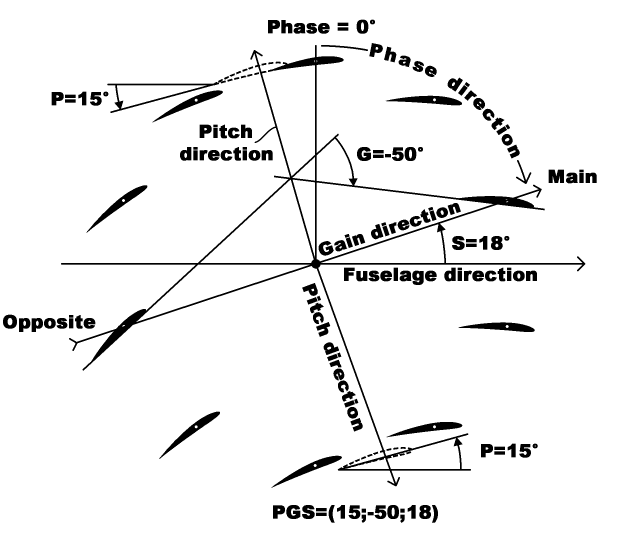# The PGS-state

Handling of the liftoplane on intermediate level is performed by steering wings on its rotors. The steering needs some system for reference a state of the steering. The system is defined here and it is referenced as PGS-state or simple PGS. The PGS definition is represented on the diagram below and follows with explanation.The diagram images wings of rotor in some particular state of steering. Main idea of it is: the state is simple cinematic characteristics, which is irrelevant to current airflow condition. And so it can be considered as low level of handling the aircraft. It can be not friendly for pilot use, but it is targeted at first only for having an exact reference. A straightforward way for it is: having number of pitches equal to number of wings. From simulation examples before we know: the aircraft can be handled by switching AoA from lifting value to recovery value and vice versa. But the AoA is a characteristic of airflow condition, which will be out of scope of desired state. Nevertheless, consider the desired state of pitches can have some symmetry correspondent to the symmetrical state of AoAs with some functional mapping between both. So if a state of AoAs can be characterized with two values in two opposed points and intermediate values between their, also the state of pitches can be characterized on same manner, where intermediate states will be reflected by some function, which will be depend from particular implementation of the steering itself and staying out of scope of referencing for the state of pitches. In such case, the referenced state will be reduced only to three parameters, where two of them will be related to two values in opposite points and third parameter will point on exact direction where these two opposite points are placed.

Additional idea there: let the system will possess some kind of neutrality in some particular cases. And it exists indeed, when pitches of all wings are equal to some value. Let use the value as first parameter of the referenced system and name it as "Pitch" with referencing by first letter "P". Next parameter will characterize a level of violation of this neutrality, which is logically connected to the difference of pitches of wings in two specific opposite points. Let reference one point "main" and other "opposite", were the word "main" is selected for reflecting its impact on much operations for lifting. And the parameter will be equal to difference in pitches between the "main" and the "opposite". And so it is second parameter, which has name "Gain" and is referenced by second letter "G". Finally, remained third parameter will be simple angular direction of the "main" point. I named the third parameter as "Skew" and reference it by third letter "S". The name I selected because there exists logical connection with the "Skew" angle for the "conveyered wings” aircraft I described in the topic about evolution in respect of implementation of the “flying elevator”conception. The aircraft also can utilize the referencing to PGS-state, although its Skew is fixed.

Now on the diagram we can see all these referenced elements. There are two Pitch directions, where wings posses the neutrality with particular "P" value. There is a Gain direction, i.e. Skew with value "S", where the second parameter "G" can be measured. And finally at bottom an example of the reference is represented as the three components vector: PGS=(15;-50;18) . Here values mean in degrees. Also it can be written as (15°;-50°;18°). Additionally the diagram references to a "Phase" parameter definition for particular wing, which is out of scope of the PGS-state, but is used for recovering actual pitch for particular wing upon substitution the entire PGS-state and the Phase to some routine, which calculates the actual pitch using particular implementation of steering functionality.